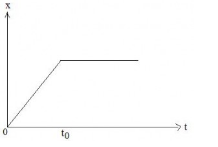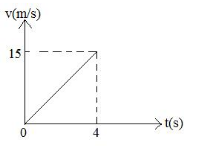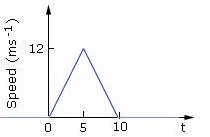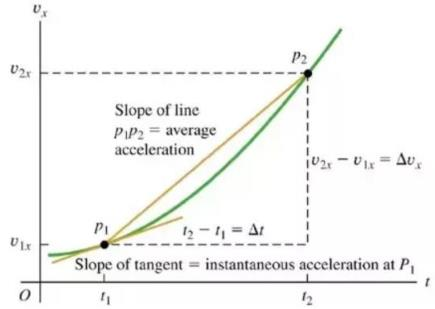Courses

# Test: Graphical Representation

## 10 Questions MCQ Test Physics Class 11 | Test: Graphical Representation

Description
This mock test of Test: Graphical Representation for JEE helps you for every JEE entrance exam. This contains 10 Multiple Choice Questions for JEE Test: Graphical Representation (mcq) to study with solutions a complete question bank. The solved questions answers in this Test: Graphical Representation quiz give you a good mix of easy questions and tough questions. JEE students definitely take this Test: Graphical Representation exercise for a better result in the exam. You can find other Test: Graphical Representation extra questions, long questions & short questions for JEE on EduRev as well by searching above.
QUESTION: 1

### Figure shows the displacement – time graph of a particle moving along X – axis.Solution:

Because velocity is the slope of the x-t graph.(dx/dt=V) and by looking at the graph it can be seen that upto time To slope is constant so velocity will be constant and after time To slope is zero ( line is parallel to X axis) so the velocity will be zero.

QUESTION: 2

### The graph represents the velocity time for the first 4 seconds of motion. The distance covered isSolution:

Calculate the area of the graph as area of v-t grph gives dis. 1/2×4×15=30

QUESTION: 3

### A person goes from his home to his office taking one hour. There he works for 2 hours and then in half hour reaches home back. A representative displacement-time graph

Solution:

We have given in the information that the person is in his office for two hours hence his position must not vary for two hours, that is the horizontal part in the graph which is only observed in the graph of option c.

QUESTION: 4

Speed time graph of a particle moving along a fixed direction is shown in the figure below. The average speed of the particle over the interval: t = 0 s to 10 s.Solution:

From the given graph we get that the area of the given graph is total distance covered that is
=  ½ x 10 x 12
=  60m
And total time taken is 10 sec
Thus average speed is  60m / 10sec
= 6 m/s

QUESTION: 5

The acceleration at any instant is the slope of the tangent of the ________ curve at that instant:

Solution:

This can be verified graphically

ObtaIning instantaneous acceleration from graphQUESTION: 6

Slope of displacement time graph or x-t graph gives us the particles’ ____________.

Solution:

Velocity is a physical vector quantity; both magnitude and direction are needed to define it. The scalar absolute value (magnitude) of velocity is called "speed", being a coherent derived unit whose quantity is measured in the SI (metric system) as metres per second (m/s) or as the SI base unit of (m⋅s−1).

QUESTION: 7

The largest numerical value is 85 and smallest numerical value is 65 and the classes desired are 8 then width of class interval is

Solution:

Total number of integral numerical values in the data are 85 - 65 = 20
Number of classes desired are 8
Hence width of each class is 20/8 = 2.5

QUESTION: 8

On velocity-time graph when two curves coincide, the objects will have

Solution:

A velocity time graph represents the velocity of an object at a very certain time. If two curves collide that means that their velocities at the given time are equal. Their acceleration may or may not be equal depending upon the slope of the curve at the point of intersection.

QUESTION: 9

Which position – time graph represents the motion of two objects that will never meet?

Solution:

In the given all options we can see that curves in graph A and D meet in the first quadrant while the curve in option b meets in the second quadrant but those of option c are parallel and hence they never meet.

QUESTION: 10

Linear inequalities are graphically represented on Cartesian plane by a

Solution:

The graph of an inequality in two variables is the set of points that represents all solutions to the inequality. A linear inequality divides the coordinate plane into two halves by a boundary line where one half represents the solutions of the inequality. The boundary line is dashed for > and < and solid for ≤ and ≥.